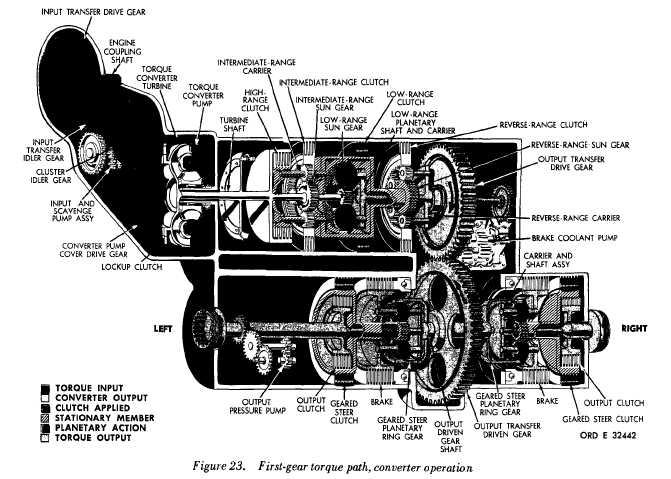FIGURE 23 FIRST GEAR TORQUE PATH, CONVERTER OPERATION
Custom SearchFigure 23 C H A P    2,   SEC   V T O R Q U E     P A T HS P A R    4   7 4 7 .    T O R Q U E    P A T H    –    F I R S T    G E A R, C O N V E R T E R    O P E R A T I O N    ( f i g .    2 3) .   In   first   gear,   torque   is   transmitted   to the   turbine   shaft   through   the   input   transfer gearing    and    the    torque    converter    (or    lockup clutch).   The   low-range   sun   gear   is   splined   to the    turbine    shaft    and    imparts    torque    to    the l o w - r a n g e     p l a n e t a r y     p i n i o n s .     T h e     l o w - r a n ge clutch   is   engaged,   holding   the   low-range   ring gear    stationary.    Thus,    the    low--range    plane- tary   pinions   must   rotate   within   the   stationary r i n g    g e a r .    T h i s    d r i v e s    t h e    l o w - r a n g e    p l a n e- tary   carrier   and   shaft   in   the   same   direction as   the   turbine   shaft   but   at   reduced   speed. .   T h e     l o w - r a n g e     c a r r i e r     s h a f t     d r i v es the    output    transfer    drive    gear.    In    turn,    the drive   gear   transmits   torque   to   the   output   trans- fer   driven   gear,   causing   it   to   rotate   in   the   op- p o s i t e    d i r e c t i o n .    T h e    d r i v e n    g e a r    t r a n s m i ts t o r q u e    t o    t h e    r i g h t -    a n d    l e f t - g e a r e d    s t e er planetary    ring    gears . .   T h e    r i g h t -    a n d    l e f t - g e a r e d    s t e e r clutches   are   engaged,   holding   the   geared   steer sun   gears   stationary.   The   geared   steer   plane - tary   pinions,   meshed   with   both   the   sun   gears and    ring    gears, a r e    f o r c e d    t o    r o t a t e    a r o u nd t h e     s t a t i o n a r y     s u n     g e a r s .     T h e     c a r r i e r     t h u s becomes   the   output   member   of   the   geared   steer planetary.   Rotation   at   reduced   speed   is   trans- mitted   to   the   right-   and   left-output   couplings. Refer   to   par.   7   for   gear   ratios   applicable   to f i r s t    g e a r. 5 0Integrated Publishing, Inc. - A (SDVOSB) Service Disabled Veteran Owned Small Business